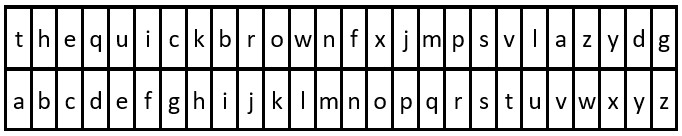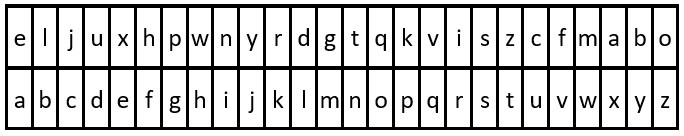2325. Decode the Message

#### QUESTION:

You are given the strings key and message, which represent a cipher key and a secret message, respectively. The steps to decode message are as follows:

Use the first appearance of all 26 lowercase English letters in key as the order of the substitution table. Align the substitution table with the regular English alphabet. Each letter in message is then substituted using the table. Spaces ‘ ‘ are transformed to themselves. For example, given key = “happy boy” (actual key would have at least one instance of each letter in the alphabet), we have the partial substitution table of (‘h’ -> ‘a’, ‘a’ -> ‘b’, ‘p’ -> ‘c’, ‘y’ -> ‘d’, ‘b’ -> ‘e’, ‘o’ -> ‘f’). Return the decoded message.

Example 1:``````Input: key = "the quick brown fox jumps over the lazy dog", message = "vkbs bs t suepuv"
Output: "this is a secret"
Explanation: The diagram above shows the substitution table.
It is obtained by taking the first appearance of each letter in "the quick brown fox jumps over the lazy dog".
``````

Example 2:``````Input: key = "eljuxhpwnyrdgtqkviszcfmabo", message = "zwx hnfx lqantp mnoeius ycgk vcnjrdb"
Output: "the five boxing wizards jump quickly"
Explanation: The diagram above shows the substitution table.
It is obtained by taking the first appearance of each letter in "eljuxhpwnyrdgtqkviszcfmabo".
``````

Constraints:

``````26 <= key.length <= 2000
key consists of lowercase English letters and ' '.
key contains every letter in the English alphabet ('a' to 'z') at least once.
1 <= message.length <= 2000
message consists of lowercase English letters and ' '.
``````

#### EXPLANATION:

easy的题目, 只要先做出字典映射, 再一一对应即可.

#### SOLUTION:

``````class Solution {
func decodeMessage(_ key: String, _ message: String) -> String {
var dic:Dictionary<Character,Character> = [:]
var result:String = ""
var index:Int = 0
for char in Array(key) {
if (char != " " && (dic[char] == nil)) {
dic[char] = Character(UnicodeScalar(97 + index)!)
index += 1
}
}
for char in message {
if char == " " {
result += String(char)
} else {
result += String(dic[char]!)
}
}
return result
}
}
``````